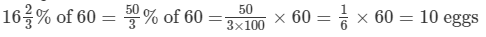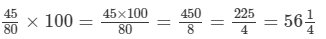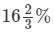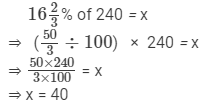Courses

# RD Sharma Solutions - Ex-11.6, Percentage, Class 7, Math Class 7 Notes | EduRev

## RD Sharma Solutions for Class 7 Mathematics

Created by: Abhishek Kapoor

## Class 7 : RD Sharma Solutions - Ex-11.6, Percentage, Class 7, Math Class 7 Notes | EduRev

The document RD Sharma Solutions - Ex-11.6, Percentage, Class 7, Math Class 7 Notes | EduRev is a part of the Class 7 Course RD Sharma Solutions for Class 7 Mathematics.
All you need of Class 7 at this link: Class 7

#### Question 1:

Ashu had 24 pages to write. By the evening, he had completed 25% of his work. How many pages were left?

Total number of pages Ashu had to write = 24
Number of pages Ashu completed by the evening = 25% of 24 = (25/100) × 24 = 600/100 = 6
Pages left for completion = 24 - 6 = 18 pages

#### Question 2:

A box contains 60 eggs. Out of whichare rotten ones. How many eggs are rotten?

Total eggs in the bag = 60
Rotten eggs =#### Question 3:

Rohit obtained 45 marks out of 80. What per cent marks did he get.

Marks obtained by Rohit = 45/80
Percentage of marks that Rohit obtained = (45/80) × 100 =#### Question 4:

Mr Virmani saves 12% of his salary. If he receives Rs 15900 per month as salary, find his monthly expenditure.

Mr. Virmani's salary = Rs. 15900 per month
Mr. Virmani's savings = 12% of Rs. 15900 = (12/100) × 15900 = Rs. 1908
Mr. Virmani's monthly expenditure = Rs. (15900 - 1908) = Rs. 13992

#### Question 5:

A lawyer willed his 3 sons Rs 250000 to be divided into portions 30%, 45% and 25%. How much did each of them inherit?

Total amount with the lawyer = Rs. 250000
First son's inheritance = 30% of 250000 = (30/100) × 250000 = 7500000/100 = Rs. 75000
Second son's inheritance = 45% of 250000 = (45/1000) × 250000 = 11250000/100 = Rs. 112500
Third son's inheritance = 25% of 250000 = (25/100) × 250000 = 6250000/100 = Rs. 62500

#### Question 6:

Rajdhani College has 2400 students, 40% of whom are girls. How many boys are there inthe college?

Total number of students in Rajdhani College = 2400
Number of girls = 40%  of 2400 = (40/100) × 2400 = 96000/100 = 960
Number of boys = 2400 - 960 = 1440 boys

#### Question 7:

Aman obtained 410 marks out of 500 in CBSE XII examination while his brother Anish gets 536 marks out of 600 in IX class examination. Find whose performance is better?

Aman's marks in CBSE XII = 410/500
Percentage of marks obtained by Aman = (410/500) ×  100 = 82

Anish's marks in CBSE IX = 536/600
Percentage of marks obtained by Anish = (536/600)  ×  100 = 89.33

So, Anish's performance (89.33%) is better than Aman's (82%).

#### Question 8:

Rahim obtained 60 marks out of 75 in Mathematics. Find the percentage of marks obtained by Rahim in Mathematics.

Marks obtained by Rahim in mathematics = 60/75
Percentage of marks obtained by Rahim  = (60/75) × 100 = 80
That is, 80%.

#### Question 9:

In an orchard,of the trees are apple trees. If the number of trees in the orchard is 240, find the number of other type of trees in the orchard.

Let the number of apple trees be 'x'.
Number of trees in the orchard = 240
Number of apple trees =of x

According to the given condition,Number of other types of trees = Total number of trees - number of apple trees = 240 - 40 = 200 trees

#### Question 10:

Ram scored 553 marks out of 700 and Gita scored 486 marks out of 600 in science. Whose performance is better?

Marks scored by Ram = 553/700
Percentage of marks scored by Ram  = (553/700) × 100 = 0.79 × 100 = 79

Marks scored by Gita = 486/600
Percentage of marks scored by Gita = (486/600) × 100 = 0.81 × 100 = 81

Gita's performance (81%) is better than Ram's (79%).

#### Question 11:

Out of an income of Rs 15000, Nazima spends Rs 10200. What per cent of her income does she save?

Nazima's total income = Rs. 15000
Amount Nazima spends = Rs. 10200
Amount Nazima saves = Rs. (15000 - 10200) = Rs. 4800
Percentage of income Nazima saves = (4800/10200) × 100 = 480000/10200 = 32
Nazima saves 32% of her income.

#### Question 12:

45% of the students in a school are boys. If the total number of students in the school is 880, find the number of girls in the school.

Total number of students in the school = 880
Number of boys in the school = 45% of 880
=  (45/100) × 880
= 39600/100 = 396
Number of girls in the school = 880 - 396 = 484

#### Question 13:

Mr. Sidhana saves 28% of his income. If he saves Rs 840 pr month, find his monthely income.

Let Mr. Sidhana's monthly  income be x.
Monthly savings = Rs. 840
28% of x = Rs. 840
⇒ (28/100)  × x = Rs. 840
⇒ 28x = Rs. 84000
⇒ x = Rs. (84000/28) = Rs. 3000
Mr. Sidhana's monthly income = Rs. 3000

#### Question 14:

In an examination, 8% of the students fail. What percentage of the students pass? If 1650 students appeared in the examination, how many passed?

Total number of students who appeared for the examination = 1650
Number of students who failed = 8% of 1650
= (8/100) ×1650
= (8 × 1650)/100
= 13200/100 = 132
Number of students who passed = 1650 - 132 = 1518
Percentage of students who passed = (1518/1650) × 100 = 0.92 × 100 = 92
That is, 92% of the students passed the examination.

#### Question 15:

In an examination, 9% of the candidates passed and 46 failed. How many candidates appeared?

Let the total number of candidates be x.
Number of candidates who failed = 46
Number of candidates who passed = 92% of
According to the given condition,
92% of x = x - 46
⇒ (92/100) x = x - 46
⇒  92x = 100x - 4600
⇒  -8x = - 4600
⇒ x = 4600/8 =
Number of candidates who appeared for the examination = 575

97 docs

,

,

,

,

,

,

,

,

,

,

,

,

,

,

,

,

,

,

,

,

,

,

,

,

,

,

,

,

,

,

;